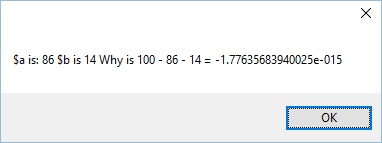## Recommended Posts

Hi,

I am using the Number function to parse a text file a translate the numbers into Integers. I have run in to a problem with the number presented on the screen are not the same as those in memory. I assume this has something do with how double is represented but should it be like this?

```for \$i = 0 to 100000 step 1
\$a = Number("0.86",3)*100
\$b = Number("0.14",3)*100
\$f = 100 - \$a - \$b
if \$f < 0 Then
msgbox(0, "", "\$a is: " & \$a & " \$b is " & \$b & " Why is 100 - " & \$a & " - " & \$b & " = " & \$f)
ExitLoop
EndIf

Next
MsgBox(0, "", "DONE!")```##### Share on other sites

Your asumption is correct. No matter how many bits are used, any binary floating point represenation of real numbers will have fiinite accuracy, so there will be gaps between representable numbers, and the size of these gaps will expand with distance from zero. Some background info is here.

`Consolewrite((.14*100)-(.15*100) & @CRLF)`

You can however use functions such as Int, Round, Floor, and Ceiling to control the result of computations, despite using real numbers as inputs.

My Contributions and Wrappers

Spoiler

## Create an account

Register a new account

• ### Recently Browsing   0 members

×

• Wiki

• Back

• #### Beta

• Git
• FAQ
• Our Picks
×
• Create New...